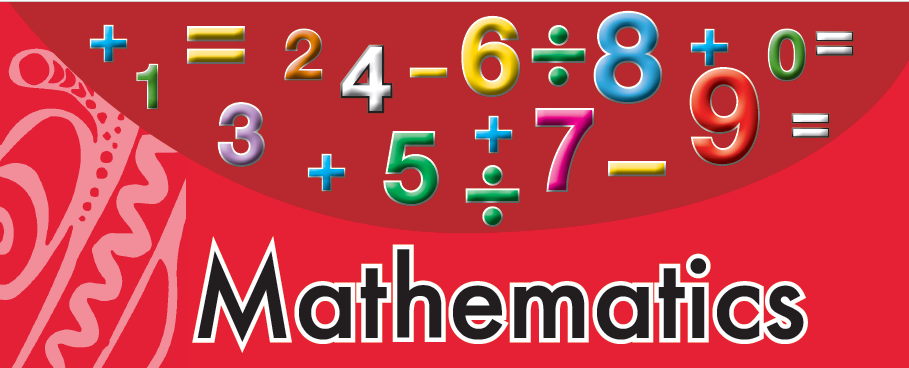# Grade 3 Mathematics Content - SBE

The Mathematics Content for Grade 3 in the PNG Standard Based Education (SBE)  as outlined in the  Standard Base Curriculum (SBC) are stated below.

Parents  can prepare their kids to master the following content for the Grade 3 level .

Teachers who are teaching at Grade 3 level should deliver the content outlined in the units below in a year.Graphics by CDD

Below are the units in mathematics that are covered at the Grade 3 level in the SBC Curriculum

• Unit 1. Addition and Subtraction 1
• Unit 2. Addition and Subtraction 2
• Unit 3. Multiplication 1
• Unit 4. Multiplication 2
• Unit 5. Thinking about How to Calculate
• Unit 6. Duration and Time
• Unit 7. Multiplication in Vertical Form
• Unit 8. Division
• Unit 9. Division with Remainders
• Unit 10 Circles and Spheres
• Unit 11 Large Numbers
• Unit 12 Length
• Unit 13 Triangles
• Unit 14 Tables and Graphs
• Unit 15 Multiplication of 2-digit Numbers
• Unit 16 Weight
• Unit 17 Fractions
• Unit 18 Math Sentences Using numbers
• Unit 19 Using Money in our life
• Unit 20 Summary of Grade 3

Also check out for

• Grade 4 Mathematics Content  - SBE
• Grade 5 Mathematics Content  - SBE
• Grade 6 Mathematics Content  - SBE
• Grade 7 Mathematics Content  - SBE
• Grade 8 Mathematics Content  - SBE
• Grade 9 Mathematics Content  - SBE
• Grade 10 Mathematics Content  - SBE
• Grade 11 Mathematics Content  - SBE
• Grade 12 Mathematics Content  - SBE
#PNGONLINESCHOOL